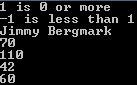## Lambda expressions and mapcar with VB.NET in VS 2008

I've started to work with Visual Studio 2008 and it's great to see that lambda expressions are supported, something I'm familiar with using in AutoLISP.

I also made a simple .NET variant of the mapcar function. If you have another way or better way let me know.

```Module Module1
Sub Main()
Dim f1 = Function(i, j) If(i >= j, i & " is " & j & " or more", i & " is less than " & j)
Console.WriteLine(f1(1, 0))
Console.WriteLine(f1(-1, 1))
Dim s1 = Function(x, y) x + " " + y
Console.WriteLine(s1("Jimmy", "Bergmark"))

Dim res() As Object = mapcar(Function(x) x * 2, New Integer() {35, 55, 21, 30})
For Each r In res
Console.WriteLine(r)
Next

Dim mult As Func(Of Integer, Func(Of Integer, Integer)) = Function(x As Integer) Function(y As Integer) x * y
Dim mult_2 = mult(2)
Dim r1 = mult_2(4)
' r1 is now 8

End Sub

Function mapcar(ByVal f As Func(Of Object, Object), ByVal ParamArray x() As Object) As Array
Dim res(UBound(x(0))) As Object
For i As Integer = 0 To UBound(x(0))
res(i) = f(x(0)(i))
Next i
Return res
End Function
End Module```

Here is the output of the code.Subscribe to the comments feed